A test!

# Doxygen index test¶

struct

Public Members

double mnp::norm_params::gamma
double mnp::norm_params::alpha
double mnp::norm_params::stability
double mnp::norm_params::volume
double mnp::norm_params::temperature
axis mnp::norm_params::anisotropy_axis
struct

Public Members

double mnp::params::gamma
double mnp::params::alpha
double mnp::params::saturation_mag
double mnp::params::diameter
double mnp::params::anisotropy
axis mnp::params::anisotropy_axis
struct

Public Functions

simulation::results::results(size_t _N)

Public Members

std::unique_ptr<double[]> simulation::results::mx
std::unique_ptr<double[]> simulation::results::my
std::unique_ptr<double[]> simulation::results::mz
std::unique_ptr<double[]> simulation::results::field
std::unique_ptr<double[]> simulation::results::time
size_t simulation::results::N
class
#include <rng.hpp>

Abstract class for random number generators.

Subclassed by RngArray, RngMtDownsample, RngMtNorm

Public Functions

virtual double Rng::get()
= 0

Get a single random number.

Return
a single draw from the random number generator

class
#include <rng.hpp>

Provides an Rng interface to a predefined array of numbers.

Inherits from Rng

Public Functions

RngArray::RngArray(const double *arr, size_t arr_length, size_t stride = 1)

Default constructor for RngArray.

Parameters
• _arr: a predefined array of random numbers
• _arr_length: length of the predefined array
• _stride: the number of consecutive elements to stride for each call to .get()

double RngArray::get()

Get the next (possibly stridden) value from the array.

The first call will always return the value at the 0th index. Each subsequent call will stride the array (default value is 1) and return that value. A call that extends beyond the end of the array will result in an error.

Exceptions
• std::out_of_range: when a call attempts to get a value beyond the maximum length of the predefined array.

Private Members

unsigned int RngArray::i

Internal state.

const size_t RngArray::max

Maximum number of draws available.

const double *RngArray::arr

Pointer to the predefined array of numbers.

const size_t RngArray::stride

Number of values to stride for each draw.

class
#include <rng.hpp>

Generate normally distributed values with downsampling.

Uses the Mersenne Twister to generate normally distributed random numbers. Down-samples the stream of random numbers by summing consecutive draws along each dimension. Function is usually used for generating coarse Wiener processes

Inherits from Rng

Public Functions

RngMtDownsample::RngMtDownsample(const unsigned long int seed, const double std, const size_t dim, const size_t down_factor)

Default constructor for RngMtDownsample.

double RngMtDownsample::get()

Get a single downsampled value from the random number generator.

Private Functions

void RngMtDownsample::downsample_draw()

Private Members

std::mt19937_64 RngMtDownsample::generator

A Mersenne Twister generator instance.

std::normal_distribution<double> RngMtDownsample::dist

A normal distribution instance.

int RngMtDownsample::current_dim

Stores the current state of the output dimenstion.

std::vector<double> RngMtDownsample::store

Stores consecutive random numbers.

const size_t RngMtDownsample::D

The number of dimensions required.

const size_t RngMtDownsample::F

The number of consecutive random numbers to downsample.

class
#include <rng.hpp>

Uses Mersenne Twister to generate normally distributed values.

Random numbers have a mean of zero and a user specified standard deviation.

Inherits from Rng

Public Functions

RngMtNorm::RngMtNorm(const unsigned long int seed, const double std)

Default constructor for RngMtNorm.

Parameters
• seed: seed for random number generator
• std: the standard deviation of the normally distributed numbers

double RngMtNorm::get()

Draw a single normally distributed value from the RNG.

Return
single normally distributed number

Private Members

std::mt19937_64 RngMtNorm::generator

A Mersenne twister generator instance.

std::normal_distribution<double> RngMtNorm::dist

A normal distribution instance.

namespace conf

Variables

list conf.extensions
dictionary conf.breathe_projects
string conf.breathe_default_project
list conf.templates_path
string conf.source_suffix
string conf.master_doc
string conf.project
string conf.copyright
string conf.author
string conf.version
string conf.release
language
list conf.exclude_patterns
string conf.pygments_style
todo_include_todos
string conf.html_theme
list conf.html_static_path
string conf.htmlhelp_basename
dictionary conf.latex_elements
list conf.latex_documents
list conf.man_pages
list conf.texinfo_documents
tuple conf.read_the_docs_build
string conf.SUFFIX
using_rtd_theme
html_style
dictionary conf.html_theme_options
list conf.html_theme_path
string conf.websupport2_base_url
string conf.websupport2_static_url
dictionary conf.context
html_context
namespace

Variables

const double constants::KB
const double constants::MU0
const double constants::GYROMAG
namespace convergence

Variables

tuple convergence.dt
tuple convergence.convergence_data
tuple convergence.diffs
tuple convergence.ensemble_diff
tuple convergence.A
tuple convergence.b
tuple convergence.least_square_res
list convergence.rate
dictionary convergence.results
namespace

Contains higher order functions for currying.

Functions

template <typename RET, typename FIRST>
std::function<RET()> curry::curry(std::function<RET(FIRST)> f, FIRST x, )

Curry single argument function.

Binds a function’s single argument and returns the callable function without arguments.

Return
std::function with no arguments
Parameters
• f: std::function with a single argument
• x: value of argument to bind to function

template <typename RET, typename FIRST, typename... REST>
std::function<RET()> curry::curry(std::function<RET(FIRST, REST...)> f, FIRST x, REST... rest, )

Curry multi-argument function.

Binds the multiple arguments to a std::function’s signature and returns the call without function call with no arguments

Return
std::function with no arguments
Parameters
• f: std::function with multiple arguments
• x: value of first argument to bind to function
• rest...: variable number of arguments to bind (can have any type)

template <typename RET, typename FIRST, typename... ARGS>
std::vector<std::function<RET()>> curry::vector_curry(std::function<RET(FIRST, ARGS...)> f, std::vector<FIRST> first, std::vector<ARGS>... args, )

Curry multi-argument function many times with lists of arguments.

Maps multiple vectors of function arguments to a vector of callable functions with all parameters bound. For example given the add function returns x+y and two std::vector<double> instances with xs={1,2},ys={3,4} then the vector_curry will return a std::vector<std::function<double()> > with two items. The first call will evaluate to 1+3=4 and a call to the second item will evaluate to 2+4=6.

Return
std::vector of callable functions
Parameters
• f: std::function with multiple arguments
• first: std::vector of different values for first argument
• args...: variable number of std::vectors with different values of function arguments (all vectors must be same length)

namespace

discrete orientation model for magnetic particles

Functions

void dom::transition_matrix(double *W, const double k, const double v, const double T, const double h, const double tau0)

Compute the 2x2 transition matrix for a single particle.

Assumes uniaxial anisotropy and an applied field h<1 Only valid for large energy barriers

void dom::master_equation_with_update(double *derivs, double *work, const double k, const double v, const double T, const double tau0, const double t, const double *state_probabilities, const std::function<double(double)> applied_field)

Computes master equation for particle in time-dependent field.

Computes the applied field in the z-direction at time t. Uses the field value to compute the transition matrix and corresponding master equation derivatives for the single particle.

Parameters
• derivs: master equation derivatives [length 2]
• work: vector [length 4]
• k: anisotropy strength constant for the uniaxial anisotropy
• v: volume of the particle in meter^3
• T: temperature of environment in Kelvin
• tau0: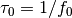where f0 is the attempt
• t: time at which to evaluate the external field
• state_probabilities: the current state probabilities for each of the 2 states (up and down) [length 2]
• applied_field: a scalar function takes a double and returns a double. Given the current time should return normalised field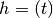where the field is normalised by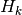namespace

Functions

void driver::rk4(double *states, const double *initial_state, const std::function<void(double *, const double *, const double)> derivs, const size_t n_steps, const size_t n_dims, const double step_size, )
void driver::eulerm(double *states, const double *initial_state, const double *wiener_process, const std::function<void(double *, const double *, const double)> drift, const std::function<void(double *, const double *, const double)> diffusion, const size_t n_steps, const size_t n_dims, const size_t n_wiener, const double step_size, )
void driver::heun(double *states, const double *initial_state, const double *wiener_process, const std::function<void(double *, const double *, const double)> drift, const std::function<void(double *, const double *, const double)> diffusion, const size_t n_steps, const size_t n_dims, const size_t n_wiener, const double step_size, )
namespace equilibrium

Functions

sw()
boltz()

Variables

tuple equilibrium.mz
tuple equilibrium.theta
tuple equilibrium.config
tuple equilibrium.t
tuple equilibrium.analytic
namespace

Contains functions for computing magnetic fields.

Functions for computing effective fields from anistoropy and a range of time-varying applied fields.

Author
Oliver Laslett
Date
2017

Functions

double field::constant(const double h, const double t)

A constant applied field.

A simple placeholder function representing a constant field. Always returns the same value.

Return
the constant field amplitude at all values of time
Parameters
• h: applied field amplitude
• t: time (parameter has no effect)

double field::sinusoidal(const double h, const double f, const double t)

A sinusoidal alternating applied field.

Returns the value of a sinusoidally varying field at any given time.

Return
the value of the varying applied field at time t
Parameters
• h: applied field amplitude
• f: applied field frequency
• t: time

double field::square(const double h, const double f, const double t)

A square wave switching applied field.

An alternating applied field with a square shape centred around zero. i.e. it alternates between values -h and h

Return
the value of the square wave applied field at time t
Parameters
• h: applied field amplitude
• f: applied field frequency
• t: time

double field::square_fourier(const double h, const double f, const size_t n_compononents, double t)

A square wave applied field of finite Fourier components.

An approximate square wave is computed from a finite number of Fourier components. The square wave alternates between -h and h.

Return
the value of the square wave applied field at time t
Parameters
• h: applied field amplitude
• f: applied field frequency
• n_components: number of Fourier components to compute
• t: time

void field::uniaxial_anisotropy(double *h_anis, const double *magnetisation, const double *anis_axis)

Effective field contribution from uniaxial anisotropy.

The effective field experienced by a single particle with a uniaxial anisotropy.

Parameters
• h_anis: effective field [length 3]
• mag: the magnetisation of the particle of [length 3]
• axis: the anisotropy axis of the particle [length 3]

void field::uniaxial_anisotropy_jacobian(double *jac, const double *axis)

Jacobian of the uniaxial anisotropy effective field term.

The Jacobian of a particle’s uniaxial anisotropy with respect to it’s magnetisation value.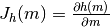Parameters
• jac: the jacobian of the effective field [length 3*3]
• axis: the anisotropy axis of the particle [length 3]

namespace

Functions

void integrator::rk4(double *next_state, double *k1, double *k2, double *k3, double *k4, const double *current_state, const std::function<void(double *, const double *, const double)> derivs, const size_t n_dims, const double t, const double step_size, )
void integrator::heun(double *next_state, double *drift_arr, double *trial_drift_arr, double *diffusion_matrix, double *trial_diffusion_matrix, const double *current_state, const double *wiener_steps, const std::function<void(double *, const double *, const double)> drift, const std::function<void(double *, const double *, const double)> diffusion, const size_t n_dims, const size_t wiener_dims, const double t, const double step_size, )
void integrator::eulerm(double *states, double *diffusion_matrix, const double *initial_state, const double *wiener_process, const std::function<void(double *, const double *, const double)> drift, const std::function<void(double *, const double *, const double)> diffusion, const size_t n_dims, const size_t n_wiener, const double t, const double step_size, )
template <class CSTATES, class CDIFF>
void integrator::milstein(CSTATES &next_state, const CSTATES &current_state, const CSTATES &drift, const CDIFF &diffusion, const CSTATES &wiener_increments, const double step_size)
int integrator::implicit_midpoint(double *x, double *dwm, double *a_work, double *b_work, double *adash_work, double *bdash_work, double *x_guess, double *x_opt_tmp, double *x_opt_jac, lapack_int *x_opt_ipiv, const double *x0, const double *dw, const std::function<void(double *, double *, double *, double *, const double *, const double, const double)> sde, const size_t n_dim, const size_t w_dim, const double t, const double dt, const double eps, const size_t max_iter, )
namespace

Functions

template <typename T>
int io::write_array(const std::string fname, T const *arr, const size_t len)
namespace

Functions for evaluating the Landau-Lifshitz-Gilbert equation.

Includes the basic equation as well as Jacobians and combined functions to update fields during integration.

Author
Oliver Laslett
Date
2017

Functions

void llg::drift(double *deriv, const double *state, const double time, const double alpha, const double *heff)

Deterministic drift component of the stochastic LLG.

Parameters
• deriv: drift derivative of the deterministic part of the stochastic llg [length 3]
• state: current state of the magnetisation vector [length 3]
• t: time (has no effect)
• alpha: damping ratio
• the: effective field on the magnetisation [length 3]

void llg::drift_jacobian(double *deriv, const double *state, const double time, const double alpha, const double *heff, const double *heff_jac)

Jacobian of the deterministic drift component of the stochastic LLG Since, in the general case, the effective field is a function of the magnetisation, the Jacobian of the effective field must be known in order to compute the Jacobian of the drift component.

Parameters
• jac: Jacobian of the drift [length 3x3]
• m: state of the magnetisation vector [length 3]
• t: time (has no effect)
• a: damping ratio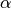• h: effective field acting on the magnetisation [length 3]
• hj: Jacobian of the effective field evaluated at the current value of m [length 3x3]

void llg::diffusion(double *deriv, const double *state, const double time, const double sr, const double alpha)

The stochastic diffusion component of the stochastic LLG.

Parameters
• deriv: diffusion derivatives [length 3x3]
• state: current state of the magnetisation [length 3]
• t: time (has no effect)
• sr: normalised noise power of the thermal field (see notes on LLG normalisation for details)
• alpha: damping ratio

void llg::diffusion_jacobian(double *jacobian, const double *state, const double time, const double sr, const double alpha)

Jacobian of the stochastic diffusion component of the LLG.

Parameters
• jacobian: Jacobian of the LLG diffusion [length 3x3]
• state: current state of the magnetisation vector [length 3]
• t: time (has no effect)
• sr: normalised noise power of the thermal field (see notes on LLG normalisation for details)
• alpha: damping ratio

void llg::sde_with_update(double *drift, double *diffusion, double *heff, const double *current_state, const double drift_time, const double diffusion_time, const double *happ, const double *anisotropy_axis, const double alpha, const double noise_power)

Computes drift and diffusion of LLG after updating the field.

The effective field is first computed based on the applied field and current state of the magnetisation. This is then used to compute the current drift and diffusion components of the LLG. Assumes uniaxial anisotropy.

Parameters
• drift: deterministic component of the LLG [length 3]
• diffusion: stochastic component of the LLG [length 3x3]
• heff: effective field including the applied field contribution [length 3]
• state: current state of the magnetisation [length 3]
• a_t: time at which to evaluate the drift
• b_t: time at which to evaluate the diffusion
• happ: the applied field at time a_t [length 3]
• aaxis: the anisotropy axis of the particle [length 3]
• alpha: damping ratio
• sr: normalised noise power of the thermal field (see notes on LLG normalisation for details)

void llg::jacobians_with_update(double *drift, double *diffusion, double *drift_jac, double *diffusion_jac, double *heff, double *heff_jac, const double *current_state, const double drift_time, const double diffusion_time, const double *happ, const double *anisotropy_axis, const double alpha, const double noise_power)

Computes effective field, drift, diffusion and Jacobians of LLG.

The effective field is first computed based on the applied field and current state of the magnetisation. This is then used to compute the drift, diffusion, and their respective Jacobians. Assumes uniaxial anisotropy.

Parameters
• drift: deterministic component of the LLG [length 3]
• diffusion: stochastic component of the LLG [length 3x3]
• drift_jac: Jacobian of the deterministic component [length 3x3]
• diffusion_jac: Jacobian of the diffusion component [length 3x3x3]
• heff: effective field including the applied field contribution [length 3]
• heff_jac: Jacobian of the effective field [length 3x3]
• state: current state of the magnetisation [length 3]
• a_t: time at which to evaluate the drift
• b_t: time at which to evaluate the diffusion
• happ: the applied field at time a_t [length 3]
• aaxis: the anisotropy axis of the particle [length 3]
• alpha: damping ratio
• s: normalised noise power of the thermal field (see notes on LLG normalisation for details)

namespace

Typedefs

using mnp::axis = typedef std::array<double,3>
namespace

Functions

void moma_config::validate_for_llg(const json input)

Validate that fields for llg simulation are in json.

Inspects a json file to check that it has the right structure and that parameters match assumptions required for simulation (e.g. temperature is not negative). Fatal logs on failure.

Parameters
• input: json config object

void moma_config::validate_for_dom(const json input)

Validate that fields for discerete-orientation-model simulation are in json.

Inspects a json file to check that it has the right structure and that parameters match assumptions required for simulation (e.g. temperature is not negative). Fatal logs on failure.

Parameters
• input: json config object

json moma_config::transform_input_parameters_for_llg(const json input)
json moma_config::transform_input_parameters_for_dom(const json input)
int moma_config::write(const std::string fname, const json output)
void moma_config::launch_simulation(const json input)

Main entry point for launching simulations from json config.

Runs simulations specified by the json configuration. The config must be normalised moma_config::normalise. Function does not return anything but will write results to disk (see logs and config options).

Parameters
• norm_config: json object of the normalised configuration file

void moma_config::launch_dom_simulation(const json input)
void moma_config::launch_llg_simulation(const json input)
namespace

Functions

int optimisation::newton_raphson_1(double *x_root, const std::function<double(const double)> f, const std::function<double(const double)> fdash, const double x0, const double eps = 1e-7, const size_t max_iter = 1000, )
int optimisation::newton_raphson_noinv(double *x_root, double *x_tmp, double *jac_out, lapack_int *ipiv, int *lapack_err_code, const std::function<void(double *, double *, const double *)> func_and_jacobian, const double *x0, const lapack_int dim, const double eps = 1e-7, const size_t max_iter = 1000, )

Variables

const int optimisation::SUCCESS
const int optimisation::MAX_ITERATIONS_ERR
const int optimisation::LAPACK_ERR
namespace

Functions

struct simulation::results simulation::full_dynamics(const double damping, const double thermal_field_strength, const d3 anis_axis, const std::function<double(double)> applied_field, const d3 initial_magnetisation, const double time_step, const double end_time, Rng &rng, const bool renorm, const int max_samples, )
struct simulation::results simulation::dom_ensemble_dynamics(const double volume, const double anisotropy, const double temperature, const double tau0, const std::function<double(double)> applied_field, const std::array<double, 2> initial_mags, const double time_step, const double end_time, const int max_samples, )

Simulates a single particle under the discrete orientation model.

Simulates a single uniaxial magnetic nanoparticle using the master equation. Assumes that the anisotropy axis is aligned with the external field in the $z$ direction.

The system is modelled as having two possible states (up and down). Given the initial probability that the system is in these two states, the master equation simulates the time-evolution of the probability of states. The magnetisation in the $z$ direction is computed as a function of the states.

Return
simulation::results struct containing the results of the simulation. The x and y components of the magnetisation are always zero.
Parameters
• damping: damping ratio - dimensionless
• anisotropy: anisotropy constant - Kgm-3 ?
• temperature: temperature - K
• tau0: reciprocal of the attempt frequency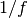- s-1
• applied_field: a scalar in-out function that returns the value of the applied field in the z-direction at time t
• initial_prbs: length 2 array of doubles with the initial probability of each state of the system.
• time_step: time_step for the integrator
• end_time: total length of the simulation
• max_samples: integer number of times to sample the output. Setting to -1 will sample the output at every time step of the integrator.

template <typename... T>
struct results simulation::ensemble_run(const size_t max_samples, std::function<results(T...)> run_function, std::vector<T>... varying_arguments, )
template <typename... T>
std::vector<d3> simulation::ensemble_run_final_state(std::function<results(T...)> run_function, std::vector<T>... varing_arguments, )
template <typename... T>
struct results simulation::steady_state_cycle_dynamics(std::function<results(d3, T...)> run_function, const int max_samples, const double steady_state_condition, const std::vector<d3> initial_magnetisations, std::vector<T>... varying_arguments, )
void simulation::save_results(const std::string fname, const struct results &res)
double simulation::power_loss(const struct results &res, double anisotropy, double magnetisation, double anisotropy_field, double field_frequency)
void simulation::zero_results(struct results &res)
namespace

Functions

void stochastic::strato_to_ito(double *ito_drift, const double *strato_drift, const double *diffusion, const double *diffusion_jacobian, const size_t n_dims, const size_t wiener_dims)
void stochastic::ito_to_strato(double *strato_drift, const double *ito_drift, const double *diffusion, const double *diffusion_jacobian, const size_t n_dims, const size_t wiener_dims)
void stochastic::master_equation(double *derivs, const double *transition_matrix, const double *current_state, const size_t dim)

Evaluates the derivatives of the master equation given a transition matrix.

The master equation is simply a linear system of ODEs, with coefficients described by the transition matrix.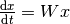Parameters
• derivs: the master equation derivatives [length dim]
• transition_matrix: the [dim x dim] transition matrix (row-major)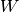• current_state: values of the state vector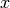namespace

Functions

double trap::trapezoidal(double *x, double *y, size_t N)
file conf.py
file conf.py
file constants.hpp
file curry.hpp
#include <functional>#include <vector>#include “curry.tpp”
file dom.hpp
#include <functional>
file field.hpp
#include <cstddef>
file integrators.hpp
#include <lapacke.h>#include <functional>
file io.hpp
#include ”../include/easylogging++.h”#include <ctime>#include <cstring>#include <cstdlib>#include <cctype>#include <cwchar>#include <csignal>#include <cerrno>#include <cstdarg>#include <string>#include <vector>#include <map>#include <utility>#include <functional>#include <algorithm>#include <fstream>#include <iostream>#include <sstream>#include <memory>#include <type_traits>
file llg.hpp
file mnps.hpp
#include <array>
file moma_config.hpp

Typedefs

using
file optimisation.hpp
#include <functional>#include <lapacke.h>
file rng.hpp
#include <random>
file simulation.hpp
#include <memory>#include <functional>#include <array>#include <random>#include <string>#include <cstdlib>#include <vector>#include “rng.hpp”#include “simulation.tpp”

Typedefs

using
using
file stochastic_processes.hpp
#include <cstdlib>
file trap.hpp
#include <cstdlib>
file dom.cpp
#include ”../include/dom.hpp”#include ”../include/constants.hpp”#include ”../include/stochastic_processes.hpp”#include <cmath>
file field.cpp
#include ”../include/field.hpp”#include <cmath>

Defines

_USE_MATH_DEFINES
file integrators.cpp
#include ”../include/integrators.hpp”#include ”../include/optimisation.hpp”#include <cmath>

Typedefs

using
using
file llg.cpp
#include ”../include/llg.hpp”#include ”../include/field.hpp”
file moma_config.cpp
#include ”../include/moma_config.hpp”#include <cmath>#include <array>#include <string>#include <omp.h>#include <exception>#include ”../include/constants.hpp”#include ”../include/easylogging++.h”#include ”../include/field.hpp”#include ”../include/simulation.hpp”#include ”../include/rng.hpp”

Defines

_USE_MATH_DEFINES

Typedefs

using
using
file optimisation.cpp
#include ”../include/optimisation.hpp”#include <cmath>#include <cblas.h>
file rng.cpp
#include ”../include/rng.hpp”#include <stdexcept>
file simulation.cpp
#include ”../include/simulation.hpp”
file stochastic_processes.cpp
#include ”../include/stochastic_processes.hpp”#include <cblas.h>
file trap.cpp
#include ”../include/trap.hpp”
file README.md
file main.cpp
#include <fstream>#include <array>#include <iostream>#include <random>#include <fenv.h>#include ”../include/moma_config.hpp”#include ”../include/json.hpp”#include ”../include/easylogging++.h”

Typedefs

using

Functions

INITIALIZE_EASYLOGGINGPP void print_help()
int main(int argc, char *argv[])
file convergence.cpp
#include <random>#include <functional>#include <fstream>#include <cstdlib>#include ”../include/easylogging++.h”#include ”../include/moma_config.hpp”#include ”../include/simulation.hpp”#include ”../include/stochastic_processes.hpp”#include ”../include/io.hpp”

Functions

INITIALIZE_EASYLOGGINGPP int main(int argc, char * argv[])
file equilibrium.cpp
#include <random>#include <functional>#include <fstream>#include <cstdlib>#include ”../include/easylogging++.h”#include ”../include/moma_config.hpp”#include ”../include/simulation.hpp”#include ”../include/stochastic_processes.hpp”#include ”../include/io.hpp”

Functions

INITIALIZE_EASYLOGGINGPP int main(int argc, char * argv[])
file convergence.py
file equilibrium.py
file tests.cpp
#include ”../googletest/include/gtest/gtest.h”#include <limits>#include <ostream>#include <vector>#include “gtest/internal/gtest-internal.h”#include “gtest/internal/gtest-string.h”#include “gtest/gtest-death-test.h”#include “gtest/gtest-message.h”#include “gtest/gtest-param-test.h”#include “gtest/gtest-printers.h”#include “gtest/gtest_prod.h”#include “gtest/gtest-test-part.h”#include “gtest/gtest-typed-test.h”#include “gtest/gtest_pred_impl.h”#include ”../include/easylogging++.h”#include ”../include/llg.hpp”#include ”../include/integrators.hpp”#include ”../include/io.hpp”#include ”../include/simulation.hpp”#include ”../include/json.hpp”#include ”../include/moma_config.hpp”#include ”../include/trap.hpp”#include ”../include/optimisation.hpp”#include ”../include/rng.hpp”#include ”../include/stochastic_processes.hpp”#include ”../include/field.hpp”#include ”../include/dom.hpp”#include <cmath>#include <random>#include <lapacke.h>#include <stdexcept>

Functions

INITIALIZE_EASYLOGGINGPP TEST(llg, drift)
TEST(llg, diffusion)
TEST(llg, drift_jacobian)
TEST(field, uniaxial)
TEST(field, uniaxial_jacobian)
TEST(heun, multiplicative)
TEST(io, write_array)
TEST(simulation, save_results)
TEST(heun_driver, ou)
TEST(trapezoidal_method, triangle)
json test_llg_config()
json test_dom_config()
TEST(moma_config, valid_llg_input)
TEST(moma_config, valid_dom_input)
TEST(moma_config, transform_llg)
TEST(moma_config, transform_dom)
TEST(newton_raphson, 1d_function)
TEST(newton_raphson, 1d_funtion_max_iter)
TEST(newton_raphson_noinv, 2d_function_2_sols)
TEST(newton_raphson_noinv, 2d_function_singular)
TEST(rng, mt_norm)
TEST(rng, array)
TEST(rng, array_stride_3)
TEST(rng, rng_mt_downsample)
TEST(implicit_integrator_midpoint, atest)
TEST(simulation, power_loss)
TEST(rk4, time_dependent_step)
TEST(master_equation, 3d_system)
TEST(dom, uniaxial_transition_matrix)
page todo

page deprecated

dir docs
dir /home/docs/checkouts/readthedocs.org/user_builds/moma/checkouts/add-docs--4/include
dir /home/docs/checkouts/readthedocs.org/user_builds/moma/checkouts/add-docs--4/lib
dir /home/docs/checkouts/readthedocs.org/user_builds/moma/checkouts/add-docs--4/test/plotting
dir /home/docs/checkouts/readthedocs.org/user_builds/moma/checkouts/add-docs--4/src
dir /home/docs/checkouts/readthedocs.org/user_builds/moma/checkouts/add-docs--4/test
page index

A **M**odern **o**pen-source **m**agnetics simulation package.. **a**gain.

Simulate magnetic nano-particles with ease.

Features

• Stochastic Landau-Lifshitz-Gilbert equation
• Explicit Heun scheme integration
• OpenMP at the highest level. Never be ashamed of embarrassingly parallel
• Json for config files and output
• Real life logging!
• You won’t believe this is C++

Join the chat at:

Installation

Moma requires LAPACK and BLAS routines. Run the [makefile](makeflie) with intel complier:

 shell $make  Make with gcc (will need version >=4.9):  shell$ make CXX=g++-4.9 

Mac OSX

The easiest way to obtain LACPACK/BLAS is through homebrew by install openblas, which comes with both. Run the following command:

 shell $brew install homebrew/science/openblas  You’ll need to link to link to the libraries when running the make command, e.g:  shell$ make CXX=g++-4.9 LDFLAGS=-L/usr/local/opt/openblas/lib CXXFLAGS=-I/usr/local/opt/openblas/include 

Tests

The fast unit tests can be run with

 shell $make tests$ ./test 

The full test suite includes numerical simulations of convergence. These take a long time to execute (~5mins). Test suite results are available in test/output.

 shell $make run-tests$ cd test/output 

Configuration

See the example config file for an overview of the options.

Dependencies

LAPACK: On Ubuntu:

 shell \$ sudo apt install liblapack-dev